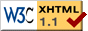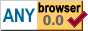Data Compression

# Data Compression

2023-03-22

## Introduction

Data compression is the art of encoding data in such a way that it takes up less space than it did originally. On this page, I briefly discuss a number of effective data compression techniques, with links to more detailed articles on each. The techniques covered here include backreferences (sliding window / dictionary compression, Lempel-Ziv), range coding, and tANS.

## Backreferences

One of the most commonly used techniques in data compression is to use backreferences to avoid repeating a sequence that has already occurred in the data. Instead of repeating the same sequence of bytes, a shorter sequence is emitted that tells the decoder to repeat the bytes it has previously seen. For example,

`Attention, attention!`

May be shortened to

`Attention, a{8, 11}!`

where {8, 11} denotes copy 8 characters, starting 11 characters before the last one. This type of compression is often referred to as sliding-window compression, dictionary compression, or Lempel-Ziv compression (after the authors of the 1977 paper titled A Universal Algorithm for Sequential Data Compression).

## Entropy Coding

Entropy coding is based on the observation that not all possible symbols are equally frequent in the input. For example, if the input consists of 8-bit bytes representing ASCII characters, we might expect that the values 32 (space) and 101 (lowercase e) are going to occur more frequently than, say, 63 (question mark). ASCII only defines values up to 127, so bytes with values above that may not occur at all. Because of this, we can save space by using an encoding that uses shorter representations for common symbols, instead of a fixed 8 bits per symbol.

By using a variable-length symbol representation, entropy coding also allows us to have more symbols than can occur in the uncompressed input, or a number of symbols that is not a power of 2. Many data compressors make use of this by performing entropy coding of a language that consists of 256 symbols representing the 256 possible byte values, plus a number of additional symbols that represent things that are not literal bytes; for example, backreferences.

Some entropy coding schemes used in data compression are:

Huffman coding
Huffman coding, also known as prefix-free code, represents symbols by bit sequences that differ in length depending on how frequently the symbol occurs in the data. Huffman coding is used in many compression formats and is fast to encode and decode, but has the limitation that the optimum size per symbol can only be approximated down to an integer number of bits.
Range coding
Range coding (and the equivalent arithmetic coding) allows the number of bits per symbol to closely approximate the optimum. This allows for better compression than is possible with Huffman coding. The tradeoff is that range coding generally takes more time to encode and decode. More about range coding.
Table-based Asymmetric Numeral Systems (tANS)
Table-based assymetric numeral systems offer fast encoding and decoding while also allowing the number of bits per symbol to approximate the optimum with sub-bit precision. More about tANS.

## Image Compression

The techniques mentioned earlier are general data compression techniques that can be applied to any type of data. There are also techniques that are commonly used for image compression. Some of those techniques are discussed in this section.

### Discrete Cosine Transform

The Discrete Cosine Transform (DCT for short) is a part of many lossy compression formats. It is a reversible transformation between sample values (e.g. pixel data) and DCT coefficients. Bits can be discarded from the DCT coefficients while still allowing the reconstruction of data that is perceptually similar to the original input. The discrete cosine transform is used in many image, video, and audio compression formats, including JPEG, MPEG, and MP3. This article describes how the discrete cosine transform works, using image data as an example. Read more about the discrete cosine transform.

## Example Code

In addition to the articles about various compression techniques, there is a project with example implementations of various algorithms in Rust. Example uses of the algorithms are provided in the tests, which can be run with `cargo test --release`.